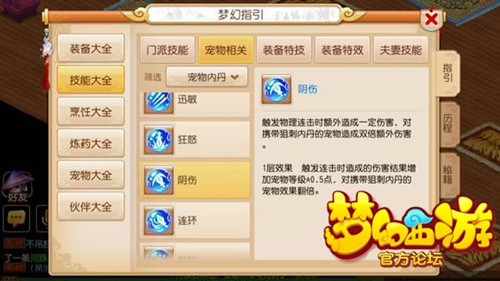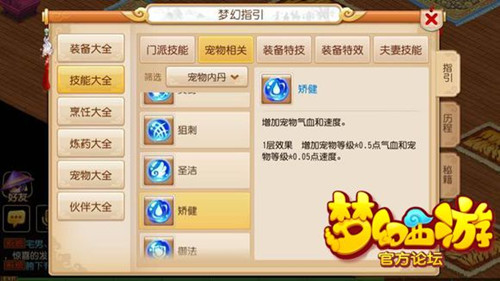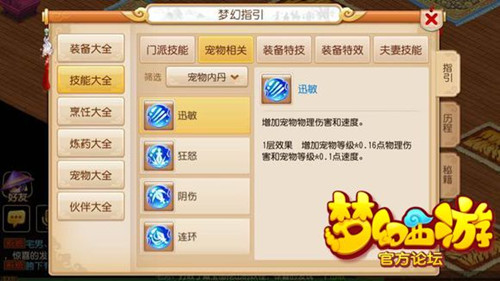# 数据帝为你详解 低级内丹之超级泡泡

因为目前主流超级泡泡都会加体  所以我当时的计算切入点是

矫健HP和速度VS迅敏攻击和速度

以矫健的HP换算成属性点 对比 迅敏的攻击换算的属性点

如果矫健高 则可以放弃迅敏改打矫健 然后少加点体

就可以把属性点加到力量上 追求属性点分配最大化优势

本文模板为 1.3成长的100级超级泡泡（即现阶段最强3升泡泡）

以官方自带模拟器估算得出体力敏对应HP攻击速度

1体=9.1HP

1力=2.04攻击

1敏=2.125速度

5级矫健

HP=等级*1=100=11个属性点=22.44攻击

速度=等级*0.1=10=4.7属性点

5级矫健=16个属性点=32.64攻击

5级迅敏

攻击=等级*0.32=32=15.7个属性点=143HP

速度=等级*0.2=20=9.4属性点

5级迅敏=25个属性点

关于迅敏补充说明：

迅敏可获取20级攻修加成，此时攻击为32*1.4=45=22属性点 加上速度的9.4属性点 则有31.4属性点

所以：（伤害角度）迅敏=22属性点，加上之前内丹篇已得出的两个结论：连环收益4%以上，奇袭收益8%

已知狂怒收益大于阴伤

先算 阴伤 连击时 等级*1=100 连击概率61% 收益61=30属性点 已大于迅敏（伤害角度）

可得伤害角度结论：狂怒收益【大于】阴伤【大于】迅敏。

迅敏的优势则在于能否破防（其实也才32点攻击）和速度加成的先出手（其实也就才20点速度）。仅此而已。

最后附送一个：

舍身击：（力量-等级）*2/5=168=82个属性点  注：模板为4力1体的成长1.3的100级超级泡泡

玄武躯：等级*2*2=400=44个属性点